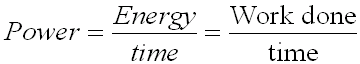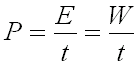#### Power

Power is the rate at which energy is used up or work is done.

Mathematically, the above statement can be expressed asIn symbols, this expression is written asand the unit of power is the    WATT (W)

1 Watt is equal to one Joule per second

1 W = 1 J/s

Example:

A crane lifts a 250 Kg crate of roof shingles from the ground on top of a 15 m high roof  in 20 s.  How much power did the crane use up?

Solution:

Given: m = 350 Kg

g = 9.8 m/s2

h = 15 m

c) Power
t =  20 s

Solution:  a) Force Required to Lift Shingles up = Fg
= m x g (the force of gravity)
= 350 Kg x 9.8 N/Kg
= 3430 N

b) Work Done = Work Done in lifting
= Fg x h
= 3430 N x 15 m
= 51 450 Nm
= 51 450 J

c) Power = Work / time
= W / t
= 51 450 J /  20 s
= 2573 W